# Catch up on the latest AI articles# Time Series Anomaly Detection Starting From Unsupervised (NCAD)

3 main points
✔️ A powerful framework is proposed for time series anomaly detection
✔️ Combines data augmentation with an expressive representation model, not just a prediction model
✔️ Does not waste even a small amount of labeled data but incorporates more labels into the model to improve performance

The images used in this article are from the paper or created based on it.

## first of all

This is a paper by AWS AI Labs. We propose NCAD (Neural Contextual Anomaly Detection), a framework for time series anomaly detection that can handle unsupervised and supervised, seamlessly, univariate and multivariate.

Unsupervised learning on a data set with few labels is inefficient because the available information is not used. Therefore, we have prepared a framework that can seamlessly handle unsupervised, semi-supervised, and supervised learning, and a structure that can incorporate additional data.

Recent developments in deep anomaly detection in computer vision have achieved remarkable performance with such a concept, a notable example being the work coupled to hypersphere classifiers, which extends one-class classification into a powerful framework for semi-supervised learning anomaly detection.

Recent developments in representation learning for multivariate time series have been realized by efficiently combining techniques originally used for anomaly detection in image processing, such as HSC (Hypersphere Classifier) and OE (Outlier Exposure), with modifications for time series. By injecting the synthesized outliers into the data at hand, it facilitates learning of the boundary between normal and outlier values. All information can be used efficiently as domain knowledge or as training labels for semi-supervised learning.

This method divides the time series data into overlapping windows of fixed length. Each window is further divided into two parts: a context window and a suspect window (Fig. 1). The goal is to detect anomalies in the suspect window. Based on the intuition that outliers induce large perturbations in the embedding, the outliers are identified in the learned latent representation space. That is, we expect the representations of two overlapping segments containing normal and abnormal values, respectively, to be far apart when compared.

Time series dissimilarity is inherently contextual. Using this, we extend HSC loss to contextual hypersphere loss. It is a dynamic fit to the center of the hypersphere by contextual representation. To facilitate the learning of the boundary between normal and abnormal, we use data expansion. Among other things, we use OE variance to create contextual dissimilarities and use simply injected outlier point dissimilarities.

## related research

We classify the previous approaches to time series anomaly detection into three categories: 1) prediction approaches, 2) recovery models and 3) compression-based approaches.

Forecasting approaches include traditional methods such as ARIMA. SPOT and DSPOT detect outliers in the time series using extreme value theory, which models the skirts of the distribution.

In deep anomaly detection, recovery model methods using VAE and GAN are derived from prediction approaches; DONUT uses VAE to predict the distribution of sliding windows. SR-CNN trains a supervised CNN on top of an unsupervised anomaly detection model, SR, using injected single outlier labels. AnoGAN uses GANs to model a sequence of observables and make probabilistic predictions in latent space. DAGMM, LSTM-VAE uses recurrent networks and VAE. OmniAnomaly extends this framework with a deep innovation state-space model and a normalization flow. MSCRED uses a convolutional self-encoder to find anomalies by measuring the recovery error. MTAD-GAT is a method using graph attention networks, which is introduced in another article.

Compression-based approaches are becoming more common in image anomaly detection. The principle is the same as the one-class classification used in the SVM-like support vector data description method SVDD (only one class in the training data). Instances are mapped to latent representations to form a sphere in the latent space. Any point away from the center of the sphere is considered an anomaly.DeepSVDD achieves this by minimizing the Euclidean distance. THOC applies this principle to the context of time series.

The Hypersphere Classifier (HSC ) is an improvement on DeepSVDD and is trained using standard binary cross-entropy. This extends it to a (semi-)supervised learning setting, where the loss of HSC is expressed in terms of pseudo-probabilities as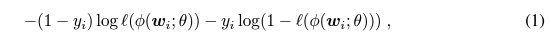Several studies have shown that remarkable performance improvements can be obtained with only a small amount of labeled outlier data. An extension of this is a powerful tool called OE (Outlier Exposure). It improves detection performance by capturing a large number of outliers from an extended dataset during training. Even though such negative examples are not true outliers, such contrasts are useful for learning property representation. Furthermore, the combination of OE and HSC has shown remarkable results in images.

For time series, artificial anomalies, and data extensions for them, have not been well studied, and SR-CNNs have been used to put unsupervised learning anomaly detection models on top of supervised learning CNNs by injecting single-point outliers.

## Model Description

The building blocks of anomaly detection are as follows Combining a window-based anomaly detection approach with a flexible learning paradigm and effective, heuristic data expansion.

Rather than predicting binary data (normal, abnormal), a positive anomaly score is predicted for each time step, followed by a threshold to satisfy the desired Precision/Recall trade-off to obtain the anomaly label.

### Window-based contextual hypersphere detection

As mentioned above, time-series data is divided into windows, each of which is further divided into a context window and a suspect window. The suspect window is usually smaller and can be a single point in time.

Anomaly detection is performed by comparing the representation vectors $\phi(w;\theta)$ and $\phi(w (C);\theta)$ of the full and context windows obtained by applying the neural network feature value extractor $\phi (\cdot ; \theta )$.

The loss function can be seen as a contextual version of the HSC.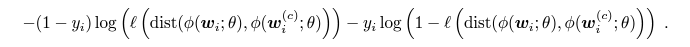Using the Euclidean distance for dist(,) and the dynamic basis function for l(), we get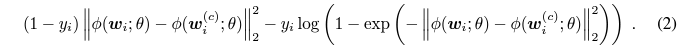Intuitively, this means that the center of the hypersphere is an HSC loss function that is dynamically chosen as a representation of the context for each context. If we learn this for generic anomaly injections, it means we can generalize it to more complex anomalies in the real world. (You can add label data)

### architecture

NCAD consists of three parts: 1) an NN encoder $\phi(\cdot ;theta)$ where we use TCN with adaptive max pooling along the time series 2) a distance-like function $dist(\cdot,\cdot)$ 3) a probabilistic score function l(z)

The encoder parameter $\theta$ is learned by minimizing the classifier loss for a mini-batch of window w.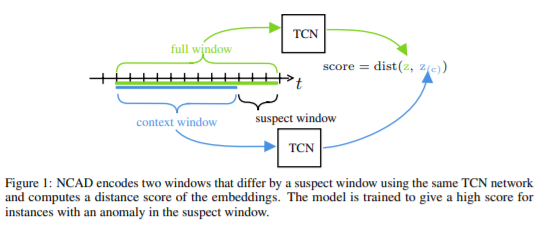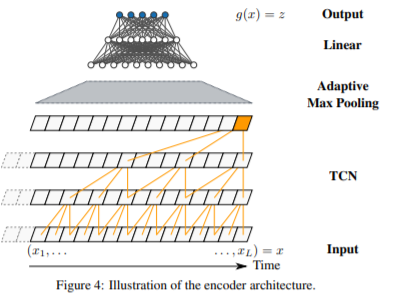In order to detect anomalies in real-time, this model is applied to a rolling window of time series data. Thus, the data at each point in time appears in multiple rolling windows. You can alarm on the first high score or on the average value.

### data extension

Another feature of this model is the use of a set of data extension methods that inject artificial outliers. The purpose of this is to allow supervised learning without using the true data (ground-truth labels). These data extension methods do not attempt to characterize the entire distribution of outliers but add an effective generic heuristic to detect common outlier cases.

Contextual Outlier Exposure (COE)

Following the success of OE, we propose a method that does not rely on the simple task of generating outlier examples in context. For the data in the Suspect window, we bring in chunks of data from other time series and replace them; Fig. 5 shows the original data, and Fig. 6 shows the data with COE applied to 1500~1550. Fig.5 shows the original data, and Fig.6 shows the data with COE applied to 1500~1550. The data are interchanged between (a) and (b), (c) and (d).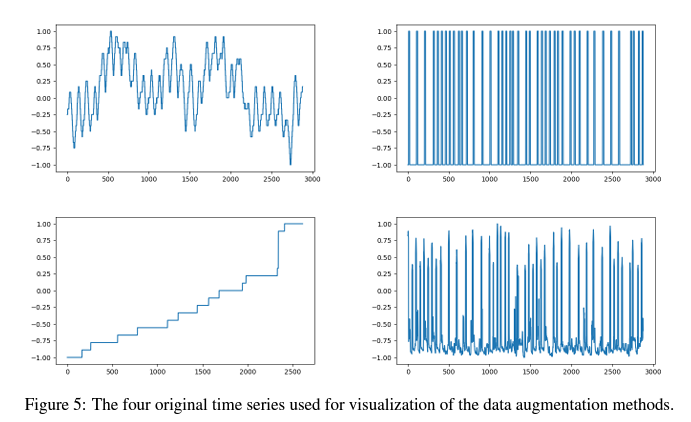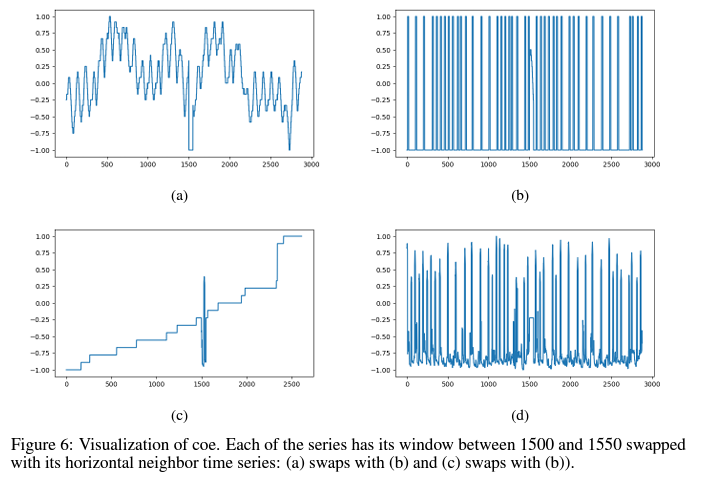Different Injection

One simple point PO(Point Outlier) is proposed in this section. We inject a spike as shown in the figure below.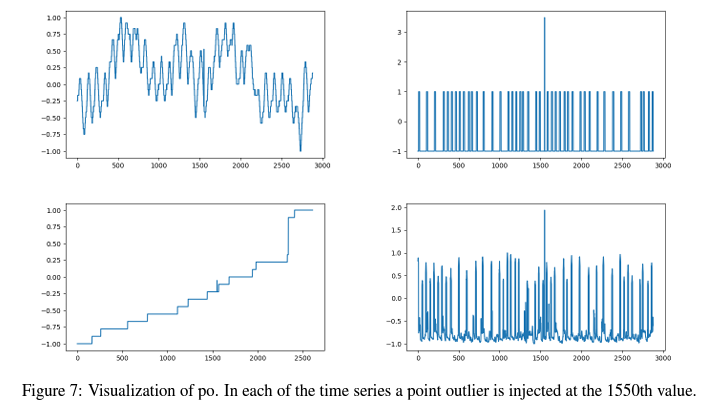Window Mixup

We perform a linear combination of the training data in a manner inspired by MIXUP. as shown in Fig. 8.